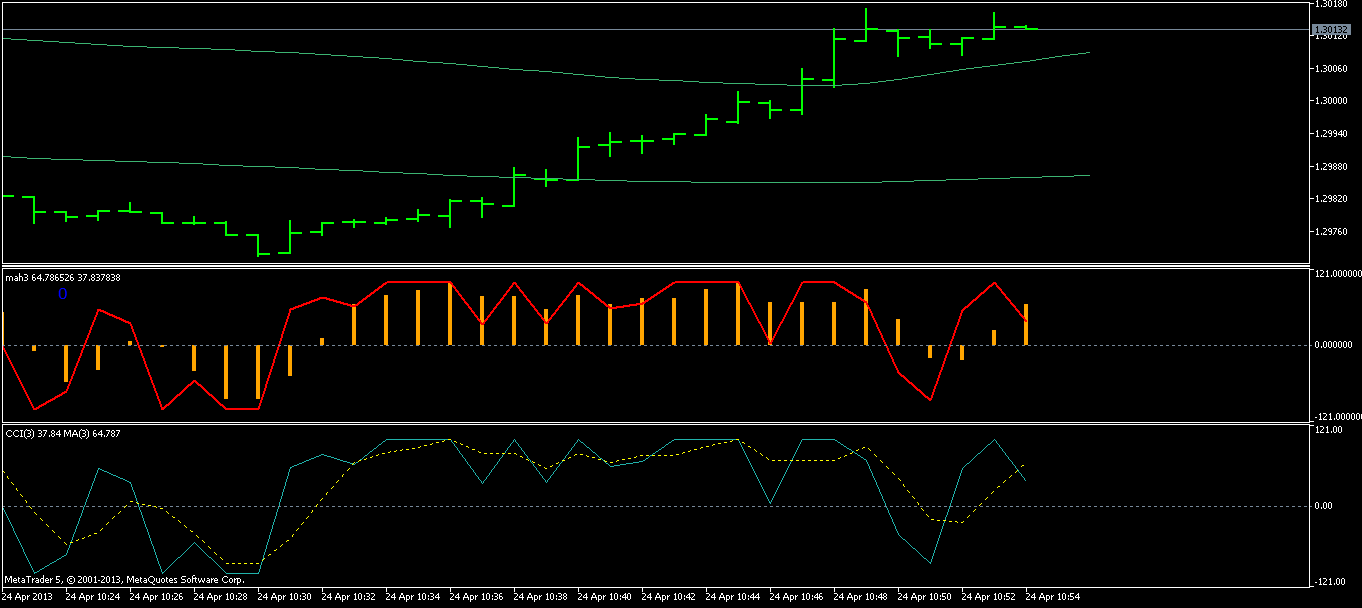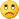# Something interesting, old thread - page 48155952

dr.house7:

I don't understand, the indicator seems react with level 0 instead of SMA...what's wrong? It should show the signal of cci crossing the sma, not the 0 level.

I tried on m1 with period1=3 and period2=3

Doc

Try out this one. It shows the moving average and CCI in the sub-window too so it can be controlled. The change is in the CopyBuffer() line

Files:
mah_2.mq5 7 kb706

Doc Try out this one. It shows the moving average and CCI in the sub-window too so it can be controlled. The change is in the CopyBuffer() line

Ok I got it, the problem is the code

if (cciValue1 > maValue1)

where obvious it's not appropriate for this and give me this problem:Sould be red signal there, but how to do in an easy method?

Files:155952

dr.house7:
Ok I got it, the problem is the code
if (cciValue1 > maValue1)

where obvious it's not appropriate for this and give me this problem:Sould be red signal there, but how to do in an easy method?

Both buffers should be set as series then in order that that comparison works as it should. Here is the corrected version

Files:
mah_3.mq5 7 kb706

this is abstract art, and you are the masterthanks706

Both buffers should be set as series then in order that that comparison works as it should. Here is the corrected version

to add more SMA with different periods, do I need to make a void? So SimpleMAOnBuffer is useless, or am I wrong?155952

dr.house7:
Another question about the code: to add more SMA with different periods, do I need to make a void? So SimpleMAOnBuffer is useless, or am I wrong?

No, you don't need to make a void

But you have to have an extra buffer (for results) for each new MA period you plan to use706

No, you don't need to make a void But you have to have an extra buffer (for results) for each new MA period you plan to use

Before wrote my last post, I did try it but without find the problemThe problem is here I think:

ArraySetAsSeries(cciValue1, true);

if(CopyBuffer (handle_cciValue1, 0, 0, 2, cciValue1) < 0)

return(0);

ArraySetAsSeries(cciValue2, true);

if(CopyBuffer (handle_cciValue2, 1, 0, 2, cciValue2) < 0)

return(0);

//-------

SimpleMAOnBuffer(rates_total, prev_calculated, period1+period2, period2, cciValue1, maValue1);

SimpleMAOnBuffer(rates_total, prev_calculated, period2+period3, period3, cciValue2, maValue2);
Files:
mah_mod.mq5 8 kb155952

dr.house7:
Before wrote my last post, I did try it but without find the problemThe problem is here I think:

ArraySetAsSeries(cciValue1, true);

if(CopyBuffer (handle_cciValue1, 0, 0, 2, cciValue1) < 0)

return(0);

ArraySetAsSeries(cciValue2, true);

if(CopyBuffer (handle_cciValue2, 1, 0, 2, cciValue2) < 0)

return(0);

//-------

SimpleMAOnBuffer(rates_total, prev_calculated, period1+period2, period2, cciValue1, maValue1);

SimpleMAOnBuffer(rates_total, prev_calculated, period2+period3, period3, cciValue2, maValue2);

It should be something like this one

Files:25

hey mladen can you modify this indicator to show a daily open line aswell? at 00:00 gmt maybe add timeshift option

Files:706

It should be something like this one

Thank you very much!

just to learn something...why here:

if(CopyBuffer (handle_cciValue1, 0, 0, (int)MathMin(rates_total-prev_calculated+2,rates_total), cciValue1) < 0) return(0); if(CopyBuffer (handle_cciValue2, 0, 0, (int)MathMin(rates_total-prev_calculated+2,rates_total), cciValue2) < 0) return(0);

copybuffer for the second has number 0?

From mql5 website:

after the indicator handle there is this: " int buffer_num, // indicator buffer number"

So I'm little confuse, cciValue2 has no. 1 so why we put 0?